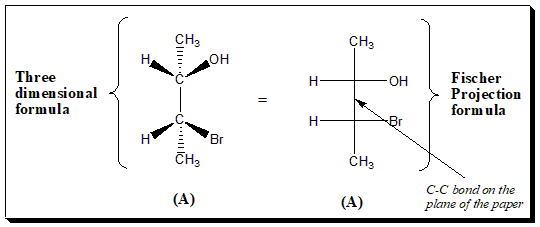## Fischer Projection: hassle free way to depict a stereoformula in 2D projection

One of the major problems in organic chemistry is the representation of three-dimensional structures in a two-dimensional media (viz. sheet of paper, blackboard, etc.). Chemists sometimes represent structures for chiral molecules with two-dimensional formulas called Fischer projection formulas. These two-dimensional formulas are a quick way to show three dimensions without the hassle of having to draw 3-D. The Fischer projection, devised by the carbohydrate chemist Emil Fischer in 1891. This projection formula is a technique …